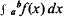# Definite Integral

(redirected from Definite Integrals)
Also found in: Dictionary, Thesaurus, Medical.
Related to Definite Integrals: Indefinite integrals
The following article is from The Great Soviet Encyclopedia (1979). It might be outdated or ideologically biased.

## Definite Integral

one of the fundamental concepts of mathematical analysis; the solution of a number of problems in geometry, mechanics, and physics reduces to a definite integral. The definite integral is a number equal to the limit of the sums of a particular type (integral sums) corresponding to a function f(x) and an interval [a, b]; it is denoted by. Geometrically, the definite integral expresses the area of a “curvilinear trapezoid” bounded by the interval [a, b] on the x-axis, the graph of the function f(x), and the ordinates of the points on the graph that have abscissas a and b. For a precise definition and generalization of the definite integral, seeINTEGRAL and INTEGRAL CALCULUS.